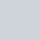# C++ Program to Find Out the Area of Square

Last updated on:

In this tutorial, we will learn about the area of square i.e. how to find out the area of square using C++

So, here’s some quick information related to the program which can help you to know better, i.e. What actually it says?

## Quick Info:💡

↪ Square is a four-sided closed figure, with equal sides each angle of 90 degree. In other words we can say that, it is a rhombus with each angle 90 degree.

↪ We are provided with the side of the square and our task is to display the result as the area of the square on the output console.

↪ Steps to perform the above task:

• First, Input the side of the square.
• Second, Use the formula to find out the area i.e. Area=Side*Side
• Third, Display the result on the output screen.

↪ It can be done using different ways:

## Find Out Area of Square Using Direct Method

Here’s a direct method to print the output on the output screen.

Simply, the user will be asked to enter the input and the area of a square is computed and displayed to a user.

## Example:

``````#include<iostream.h>
#include<conio.h>
void main()
{
int side, area;
cout<<"Enter the side of a square:";
cin>>side;
area=side*side;
cout<<"Area of a square is:";
cout<<area;
getch();
}``````
``````//Output:
Enter the side of a square:5
Area of a square is: 25``````

## Working:

Here, we have seen the simple way to find out the area of square But, now let’s try another way to find out the result of the same program.

## Find Out Area of Square Using Function

Functions are used to perform certain actions, and they are important for reusing code: Define the code once and use it many times.

Here’s the method to find out the area of square using function.

Note: Always remember that execution starts from the main body only.

### Example:

``````#include<iostream.h>
#include<conio.h>
int area_of_square(int s)
{
int a=s*s;
cout<<"Area of square for "<<s<<" is: ";
return a;
}
void main()
{
int side1,side2;
cout<<"Enter the side of a square: ";
cin>>side1;
cout<<area_of_square(side1);
cout<<"\nEnter the side of a square: ";
cin>>side2;
cout<<area_of_square(side2);
getch();
}``````
``````//Output:
Enter the side of a square: 5
Area of square for 4 is: 25
Enter the side of a square: 7
Area of square for 6 is: 49``````

### Working:

Well, I hope it really helped you to know the C++ better.

Attention reader⚠ Don’t stop learning now.

You can also try out the more program like area of rectangle, area of circle etc.

Just stay with us to get more such exciting codes to explore more in C++.

1.